University Physics Volume 2

67.

A circuit contains a D cell battery, a switch, a $20-Ω20-Ω$ resistor, and four 20-mF capacitors connected in series. (a) What is the equivalent capacitance of the circuit? (b) What is the RC time constant? (c) How long before the current decreases to $50%50%$ of the initial value once the switch is closed?

68.

A circuit contains a D-cell battery, a switch, a $20-Ω20-Ω$ resistor, and three 20-mF capacitors. The capacitors are connected in parallel, and the parallel connection of capacitors are connected in series with the switch, the resistor and the battery. (a) What is the equivalent capacitance of the circuit? (b) What is the RC time constant? (c) How long before the current decreases to $50%50%$ of the initial value once the switch is closed?

69.

Consider the circuit below. The battery has an emf of $ε=30.00Vε=30.00V$ and an internal resistance of $r=1.00Ω.r=1.00Ω.$ (a) Find the equivalent resistance of the circuit and the current out of the battery. (b) Find the current through each resistor. (c) Find the potential drop across each resistor. (d) Find the power dissipated by each resistor. (e) Find the total power supplied by the batteries.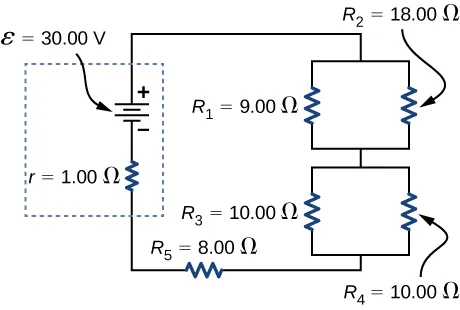70.

A homemade capacitor is constructed of 2 sheets of aluminum foil with an area of 2.00 square meters, separated by paper, 0.05 mm thick, of the same area and a dielectric constant of 3.7. The homemade capacitor is connected in series with a $100.00-Ω100.00-Ω$ resistor, a switch, and a 6.00-V voltage source. (a) What is the RC time constant of the circuit? (b) What is the initial current through the circuit, when the switch is closed? (c) How long does it take the current to reach one third of its initial value?

71.

A student makes a homemade resistor from a graphite pencil 5.00 cm long, where the graphite is 0.05 mm in diameter. The resistivity of the graphite is $ρ=1.38×10−5Ω/mρ=1.38×10−5Ω/m$. The homemade resistor is place in series with a switch, a 10.00-mF uncharged capacitor and a 0.50-V power source. (a) What is the RC time constant of the circuit? (b) What is the potential drop across the pencil 1.00 s after the switch is closed?

72.

The rather simple circuit shown below is known as a voltage divider. The symbol consisting of three horizontal lines is represents “ground” and can be defined as the point where the potential is zero. The voltage divider is widely used in circuits and a single voltage source can be used to provide reduced voltage to a load resistor as shown in the second part of the figure. (a) What is the output voltage $VoutVout$ of circuit (a) in terms of $R1,R2,andVin?R1,R2,andVin?$ (b) What is the output voltage $VoutVout$ of circuit (b) in terms of $R1,R2,RL,andVin?R1,R2,RL,andVin?$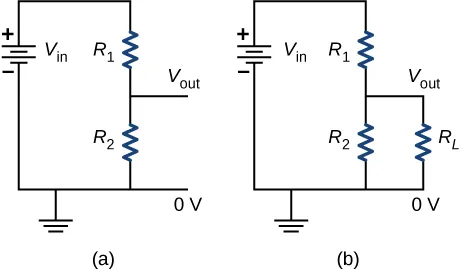73.

Three $300-Ω300-Ω$ resistors are connect in series with an AAA battery with a rating of 3 AmpHours. (a) How long can the battery supply the resistors with power? (b) If the resistors are connected in parallel, how long can the battery last?

74.

Consider a circuit that consists of a real battery with an emf $εε$ and an internal resistance of r connected to a variable resistor R. (a) In order for the terminal voltage of the battery to be equal to the emf of the battery, what should the resistance of the variable resistor be adjusted to? (b) In order to get the maximum current from the battery, what should the resistance of the variable resistor be adjusted to? (c) In order for the maximum power output of the battery to be reached, what should the resistance of the variable resistor be set to?

75.

Consider the circuit shown below. What is the energy stored in each capacitor after the switch has been closed for a very long time?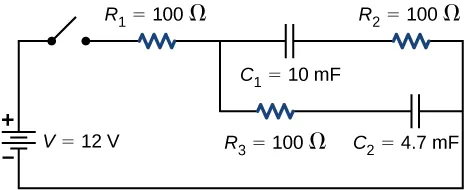76.

Consider a circuit consisting of a battery with an emf $εε$ and an internal resistance r connected in series with a resistor R and a capacitor C. Show that the total energy supplied by the battery while charging the capacitor is equal to $ε2Cε2C$.

77.

Consider the circuit shown below. The terminal voltages of the batteries are shown. (a) Find the equivalent resistance of the circuit and the current out of the battery. (b) Find the current through each resistor. (c) Find the potential drop across each resistor. (d) Find the power dissipated by each resistor. (e) Find the total power supplied by the batteries.78.

Consider the circuit shown below. (a) What is the terminal voltage of the battery? (b) What is the potential drop across resistor $R2R2$?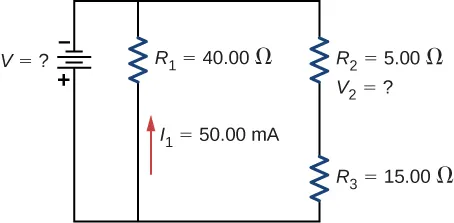79.

Consider the circuit shown below. (a)Determine the equivalent resistance and the current from the battery with switch $S1S1$ open. (b) Determine the equivalent resistance and the current from the battery with switch $S1S1$ closed.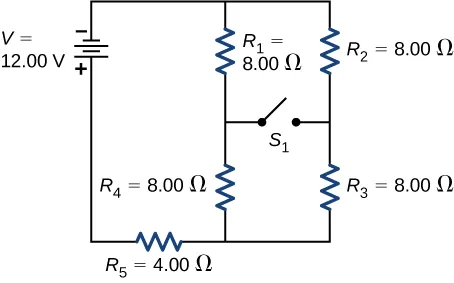80.

Two resistors, one having a resistance of $145Ω145Ω$, are connected in parallel to produce a total resistance of $150Ω150Ω$. (a) What is the value of the second resistance? (b) What is unreasonable about this result? (c) Which assumptions are unreasonable or inconsistent?

81.

Two resistors, one having a resistance of $900kΩ,900kΩ,$ are connected in series to produce a total resistance of $0.500MΩ0.500MΩ$. (a) What is the value of the second resistance? (b) What is unreasonable about this result? (c) Which assumptions are unreasonable or inconsistent?

82.

Apply the junction rule at point a shown below.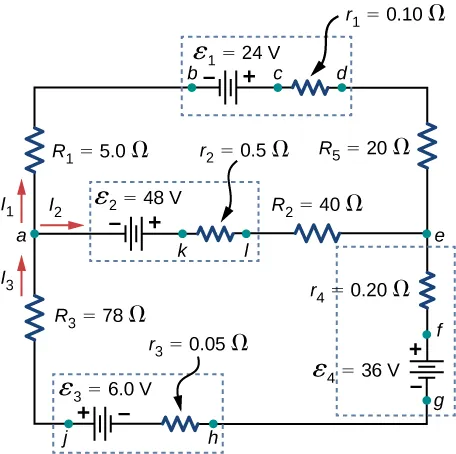83.

Apply the loop rule to Loop akledcba in the preceding problem.

84.

Find the currents flowing in the circuit in the preceding problem. Explicitly show how you follow the steps in the Problem-Solving Strategy: Series and Parallel Resistors.

85.

Consider the circuit shown below. (a) Find the current through each resistor. (b) Check the calculations by analyzing the power in the circuit.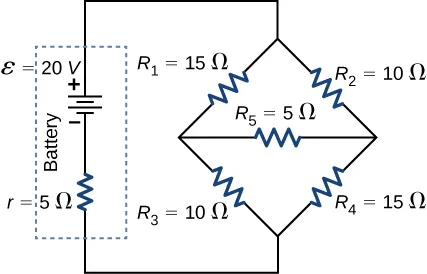86.

A flashing lamp in a Christmas earring is based on an RC discharge of a capacitor through its resistance. The effective duration of the flash is 0.250 s, during which it produces an average 0.500 W from an average 3.00 V. (a) What energy does it dissipate? (b) How much charge moves through the lamp? (c) Find the capacitance. (d) What is the resistance of the lamp? (Since average values are given for some quantities, the shape of the pulse profile is not needed.)

87.

A $160-μF160-μF$ capacitor charged to 450 V is discharged through a $31.2-kΩ31.2-kΩ$ resistor. (a) Find the time constant. (b) Calculate the temperature increase of the resistor, given that its mass is 2.50 g and its specific heat is $1.67kJ/kg·°C,1.67kJ/kg·°C,$ noting that most of the thermal energy is retained in the short time of the discharge. (c) Calculate the new resistance, assuming it is pure carbon. (d) Does this change in resistance seem significant?

Order a print copy

As an Amazon Associate we earn from qualifying purchases.